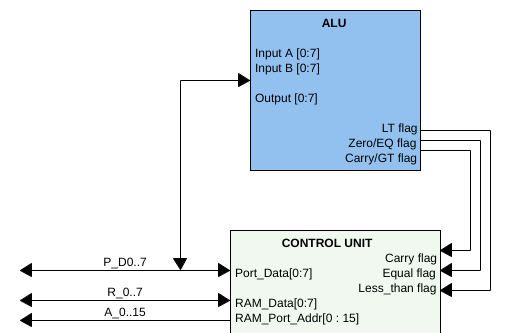# We have an ALU

We now have a port-mappable ALU. That means, the ALU is not connected directly to the CPU registers, but needs to be accessed via port I/O commands. This is indicated in the illustration below:The ALU has the following 4 registers

• Operand A
• Operand B
• Instruction
• Result

These registers need to be accessed via 4 port addresses. In my implementation I am using the ports \$fffc - \$ffff.

On top of the registers, the ALU also provides 3 flags to the CPU:

• EQ/zero: set if a comparison yielded the result ‘equal’ or if the numerical result of an operation was 0.
• GT/carry: set if a comparison yielded the result ‘greater than’ or if an addition/subtraction over/underflowed or if a shift operation shifted a bit into this flag.
• LT: set if a comparison yielded the result ‘less than’

The ALU is capable of executing the following 14 instructions:

• subtract with carry
• shift left, MSB into carry
• shift right, LSB into carry
• bitwise rotate left
• bitwise rotate right
• bitwise or
• bitwise and
• bitwise nand
• bitwise xor
• bitwise compare
• increment by 1
• decrement by 1

Some of these instructions take 2 parameters, some only one.

Here is an excerpt of `alu.inc` which defines constants and convenience macros for working with the ALU:

``````; -------------------
; -- ALU constants --
; -- for RRISC ALU --
; -------------------

const ALU_SUB  =  1    ; subtraction with carry, sets carry(gt)
const ALU_SHL  =  2    ; shift left into carry, sets carry(gt)
const ALU_SHR  =  3    ; shift right into carry, sets carry(gt)
const ALU_ROL  =  4    ; rotate left
const ALU_ROR  =  5    ; rotate right
const ALU_OR   =  6    ; binary or
const ALU_AND  =  7    ; binary and
const ALU_NAND =  8    ; binary not and
const ALU_XOR  =  9    ; binary exclusive or
const ALU_XNOR = 10    ; binary exclusive not or
const ALU_CMP  = 11    ; compare, sets carry (gt), sm, eq flags
const ALU_INC  = 12    ; increments A by 1, sets equal (zero) flag on zero
const ALU_DEC  = 13    ; decrements A by 1, sets equal (zero) flag on zero

; --
; -- port I/O mapping of ALU registers
; --
const ALU_PORT_A      = \$fffc
const ALU_PORT_B      = \$fffd
const ALU_PORT_INSTR  = \$fffe
const ALU_PORT_RESULT = \$ffff

; --
; -- ALU convenience macros
; --
out G, ALU_PORT_A
ldg # \$1
out G, ALU_PORT_B
out G, ALU_PORT_INSTR
in G, ALU_RESULT
ENDMACRO
``````

So the following assembly snippet would add a value to register G, without having to deal with ALU port operations:

``````include alu.inc   ; -- read the ALU macro definitions

org 0
ldg # \$10         ; G = 10
macro ADD_G \$20   ; instructs the ALU to add \$20 to register G
;   and to return the result in register G

; ...
``````

^ toc